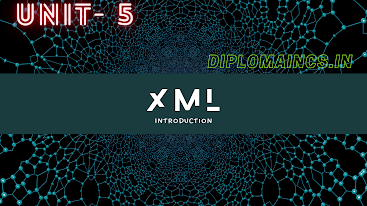## 4/7/21

### Object oriented programming c++ diploma question

This question was conducted only for LE (Letral Entry) students in the month of march -2021

(Regular student's exam were postpone due to COVID-19)

Sub Code: - 1618404

Special

2020(EVEN)

Time: 3Hrs

Semester  IV(New)/ CSE

O.O.P. Thro. C++

Full Marks: 70

Pass marks: 28

Group A

Choose the most suitable answer from the following options: -   (1*20=20)

(i) Which of the following correctly declares an array?

(a) int array ;

(b) int array;

(c) array {10};

(d) array array,

(ii) What does ‘\t’ represent?

(b) Backslash

(c) Tab

(d) Form feed

(iii) The value 132.54 can be represented using which data type?

(a) Double

(b) Void

(c) Int

(d) Bool

(iv) How many characters are specified in the ASCII scheme?

(a)64

(b) 128

(c) 256

(d)  24

(v) Which of the following will not return a value?

(a) Null

(b) Void

(c) Empty

(d) Free

(vi) The operator used for dereferencing or indirection is___________

(a) *

(b) &

(c) à

(d) à>

(vii) The constants are also called ass

(a) Const

(b) Preprocessor

(c) Literals

(d) Variables

(viii) Which operator is having the highest precedence?

(a) Postfix

(b) Unary

(c) Shift

(d) Equality

(ix) Where does the execution of the program starts?

(a) User-defined function

(b) main function

(c) void function

(d) else function

(x)  Who created C++?

(a) Bjarne Stroustrup

(b) Dennis Ritchie

(c) Ken Thompson

(d) Brian Kernigham

(xi) Which of the following is an abstract data type?

(a) Class

(b) Int

(c) Float

(d) String

(xii) Which of the following is not an entry controlled loop?

(a) For

(b) Do-while

(c) While

(d) None of these

(xiii) What does a class can hold?

(a) Data

(b) Functions

(c) Both (a) and (b)

(d) None of these

(xiv) What does inheritance allows you to do?

(a) Create a class

(b) Create a hierarchy of classes

(c) Access methods

(d) None of the mentioned

(xv) Which of the following is used to implement the C++ interfaces

(a) Absolute variables

(b) Abstract classes

(c) Constant variables

(d) None of the mentioned

(xvi) Which operator is used for input stream?

(a) >

(b) >>

(c) <

(d) <<

(xvii) An inline function is expanded during________

(a) Compile time

(b) Run - time

(c) Never expanded

(d) End of the program

(xviii) By default how the value are passes in C++?

(a) Call by value

(b) Call by reference

(c) Call by pointer

(d) Call by object

(xix) Which key word is used to define the macros in C++?

(a) Macro

(b) Define

(c) #define

(d) None of the mentioned

(xx) Which operator is used to signify the namespace?

(a) Conditional operator

(b) Ternary operator

(c) Scope operator

(d) Bitwise operator

Group:-"B"

Answer all Five Questions: -                         (5*4=20)

2. What is a function? Show how functions are defined and called with an example.

OR

What do you mean by arguments and return values in function? What is different between argument and parameter? Give example.

3.

a.          What do you mean by basic data types in C++. Explain in brief.

b.          Describe with examples any two control statements in C++.

OR

a.    What is a user defined data type? Explain with an example.

b.    How many loops are there in C++? Describe any two of them.

4.

a.          What is dynamic memory allocation (DMA)?

b.          Define the term object.

OR

a.    How to initialize a pointer?

b.    Define the term class in C++.

5.

a.    What is OOP?

b.    How OOP is different from procedural programming?

OR

a.    List four features of C++ as OOP.

b.    Do you think C++ is better than C? Justify your answer.

6. Explain the following terms: -

a.    Data abstraction

b.    Data encapsulation

OR

Write short notes on: -

b.    Polymorphism

Group:- "C"

Answer all Five Questions: -                         (5*6=30)

7. What is a constructor? Explain in brief basic, parameterized and multiple constructors.

OR

Explain the use of copy constructor?

OR

9. What are reference variables? Illustrate the passing of reference variables to function.

OR

Define inheritance.

10. What is Static function?

OR

Write short notes on: -

a.    Virtual class function

b.    Abstract class

11.

a.    What is the use of scope resolution operator?

b.    Write a C++ program to find the sum of array elements.

OR

a.    What are static variables?

b.    Write a program to find the factorial of any number using recursion.